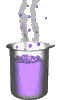Science class online
Chemistry and physics lessons

# Electricity

Free online electricity lessons for elementary school,  middle school and high school.Science class Chemistry Electricity Optics Mechanics Electricity lessons Electrical components -Two-terminal electronic components - Basic electrical components - Diodes - What's a resistor ? - How to determine a resistor value ? - Resistors effects in circuits - How to use a resistor ? - Characteristic curve of a resistor How to build simple circuits and draw diagrams - How to build a basic electrical circuit - How to draw diagrams of electric circuits The electric current - Conductors and insulators - Direction of electric current in a circuit - The dangers of electricity - Current intensity - units how to measure current ? The Voltage - The voltage and its units - How to measure a voltage ? - Voltage in open and closed circuits - Rated current and voltage for a lamp Alternating voltage and current - Alternating currents effects on led - What are alternating current and voltage ? - Periodic alternating voltage and its properties - Oscilloscope - Oscillogram - Frequency - Measuring RMS voltage with a voltmeter Serie circuits - What's a serie circuit ? - Series circuit properties - Short circuit in  series circuit - Current law in series - circuits - Voltage law in series circuits Parallel circuits - What's a parallel circuit ? - Some parallel circuits properties - Short circuits in parallel circuits - Nodes and branches in parallel circuits - Current laws in parallel circuits - Voltage laws in parallel circuits Laws of electricity - Ohm's law - Current laws in series circuits - Voltage laws in series circuits - Current laws in parallel circuits - Voltage laws in parallel circuits Generating electricity - Voltage for coil wires What is an alternator ? - Alternating currents and voltages - Generating electricity in power plants Electric power and energy - Electric power and power rating - Electric power consumption by an electrical device - Relationship between - Electric power and energy

 _____________________________________________________   _____________________________________________________

## Electric power and energy

### Electric power and power rating

 1) Voltage and current rating Voltage and current ratings are the voltage and current received by a electric component or device operating under normal conditions. When an electric device is exposed to its voltage rating then its current correspond to its current rating (and vice versa). 2) The electric power unit The electrical power is a quantity used in electricity. It is denoted with letter P and its unit is the watt (W) We also use derived units: - Kilowatt (kW):  1 ​​kW = 1000 W - The megawatt (MW): 1 MW = 1,000,000 W - The gigawatt (GW): 1 GW = 1 billion W   etc. An electric component or an electric device is also characterized by its power rating. ______________________________________ ______________________________________ 3) Relationship between electrical energy and electric power Definition: Electrical power is the energy exchanged (received or given) during a second. The power thus reflects the rate and extent with which energy is transferred or converted. 4) Meaning of power rating The more power rating is high, the more efficience is high. for instance, the more power rating is high: - the brighter a lamp is. - the more effective is suction of a vacuum cleaner. - the louder are sounds produced by speakers. Examples of power ratings: Plasma TV: 200W; iron 1 kW; washing machine 2 kW; an oven: 3 kW. If the electrical power supplied to a device is less than its power rating then its efficiency is lower than expected. If the electrical power supplied to a device exceeds its power rating then its efficiency is higher than expected but the deterioration comes faster. ______________________________________ ______________________________________
____________________________________

____________________________________